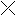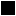## How to Play Picross

This tutorial will teach you the basic techniques for solving Picross puzzles.### Introduction

So you want to learn Picross? Follow along with the tutorial below to learn the basic techniques for solving Picross puzzles. When you're done, read through the Picross example to follow along and see how to solve a Picross puzzle.

### What is Picross?

Picross puzzles look like grids of squares with numbers above and to the left.

Each number tells you that there is group of consecutive black squares somewhere in that row or column. For example, a 5 above a column means that somewhere in that column, there is a group of five black squares with no spaces between them.

If there is more than one number for a row or column, it means that there are multiple groups of black squares, separated by at least one white square.

You must use logic to figure out which squares should be filled in, and which should stay blank. When you solve the puzzle, the grid will have a picture in it. This tutorial will teach you some Picross puzzle solving techniques.

### Empty Row, Full Row

The number to the left of this row is 0. This means that all of the squares should be white; none should be filled in. Click each white square twice to create an X, so you will know not to fill it in.

The number to the left of this row is 5, and there are five squares in the row. That means you must fill in all five squares. Click each square once until they are all black.

It might not be obvious right away, but there is only one way to fill in this row. If you add up all of the numbers, plus a 1 for a white square between each, you get 10 (1 + 1 + 4 + 1 + 3 = 10). Ten is the total number of squares in this row, so it can only be filled in one way:

### Overlap

Only 7 squares need to be filled in. Where?

When you put the 7 squares at the left side, and then the right side, the 4 middle squares are filled in both times. This means that the four squares in the middle will definitely be filled in, no matter where the other three black squares might be. Try filling in the four middle squares below.

Overlapping is one of the first strategies you should try when you start solving a puzzle. If you have trouble imagining the squares at the left side and right sides of the grid, you can put an X at the ends of where the squares will be, like this:

Marking squares with Xs like this can help you figure out where black squares should go.

Here is another example:

This example can also be filled with the overlap technique.

However, you should only fill in the one square that overlaps from the group of 4.

The squares where 2 and 4 overlap are from two different numbers, so they will not necessarily be filled in.

### On The Edge, Solve A Row

The number for this row is 2, so two squares must be filled in. You cannot use the overlap technique here, because 2 is too small a number to overlap in this row. But notice that the filled-in square is along the right side of the grid. Since you can only fill in two black squares next to each other in this row, the second one must be to the left of the filled-in one.

This row has been solved now, so to avoid confusion later, you should put Xs in the white squares. Click each white square twice to create an X there.

### Cross It Out

In this row, you can't be sure where the other two black squares should go to complete the group of four. However, you can tell which squares will never be filled in, because they are too far away to be a part of the group of four. Cross out those squares that will never be filled in.

When you cross out squares this way, be sure not to cross out squares that could still be filled in.

Here is another example:

In this example, there is already an X in the row. There are two areas where a large group of squares may go: to the left, or to the right. This row's number is 5, so there is not enough room to the left. Cross out the squares to the left of X; they will never be filled.

From there, you can use the overlap technique in the area of the six white squares.

When solving Picross puzzles, don't guess! If you get stuck with a difficult puzzle, you might be tempted to make guesses. However, you should be able to solve Picross puzzles just using logic.

If you are stuck, you might be overlooking a place where you can make progress. Keep looking, and try to use logic to figure out where a black square or an X must go.

If you do end up making guesses, keep in mind that you might end up making contradictions, and you'll have to back up and figure out where you made a mistake.

### Conclusion

Now you know the basic techniques for solving Picross puzzles. As you try to solve your first puzzles, you will learn how to use and combine these techniques to discover which squares must be filled.

### Example

The example will show you the steps taken to solve the sample puzzle at the top of this page. You don't have to solve all Picross puzzles the same way; the example is just to help give you ideas for how to solve Picross puzzles.